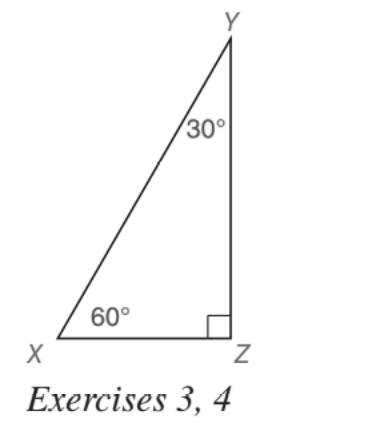Chapter 5.5, Problem 4EElementary Geometry For College St...

7th Edition
Alexander + 2 others
ISBN: 9781337614085

Solutions

Chapter
SectionElementary Geometry For College St...

7th Edition
Alexander + 2 others
ISBN: 9781337614085
Textbook Problem

For the 30 ° - 60 ° - 90 ° triangle shown, suppose that X Y = 2 a . Find: a) X Z b) Y ZTo determine

a) To find:

XZ, for the 30°-60°-90° triangle shown, suppose that XY=2a.

Explanation

Approach:

For a right triangle, for which the measure of the interior angles 30°, 60°, and 90°; if ‘a’ is the length of measure of the shorter leg; opposite to the angle 30°, then the length of the other two sides is given by

Length of the longer leg (opposite to 60°) =a3

Length of the hypotenuse (opposite to 90°)=2a.

In general

Length of the longer leg =3× (Length of the shorter leg)

Length of the hypotenuse =2× (Length of the shorter leg)

Calculation:

Given,

A 30°-60°-90° triangle XYZ with XY=2a.

30°-60°-90° theorem.

In a right triangle whose angle measure 30°, 60°, and 90°, the hypotenuse has a length equal to twice the length of the shorter leg, and the longer leg is the product of 3 and the length of the shorter leg

To determine

b) To find:

YZ, for the 30°-60°-90° triangle shown, suppose that XY=2a.

Still sussing out bartleby?

Check out a sample textbook solution.

See a sample solution

The Solution to Your Study Problems

Bartleby provides explanations to thousands of textbook problems written by our experts, many with advanced degrees!

Get Started

Find the complex conjugate and the modulus of the number. 16. 1+22i

Single Variable Calculus: Early Transcendentals, Volume I

Expand each expression in Exercises 122. (x+1)(x3)

Finite Mathematics and Applied Calculus (MindTap Course List)

83-94 Evaluate the integral. 55edx

Calculus (MindTap Course List)

In Exercises 7-10, find the slope of the line shown in each figure. 7.

Applied Calculus for the Managerial, Life, and Social Sciences: A Brief Approach

True or False: f(x) = 3x x3 is concave down for x 1.

Study Guide for Stewart's Single Variable Calculus: Early Transcendentals, 8th